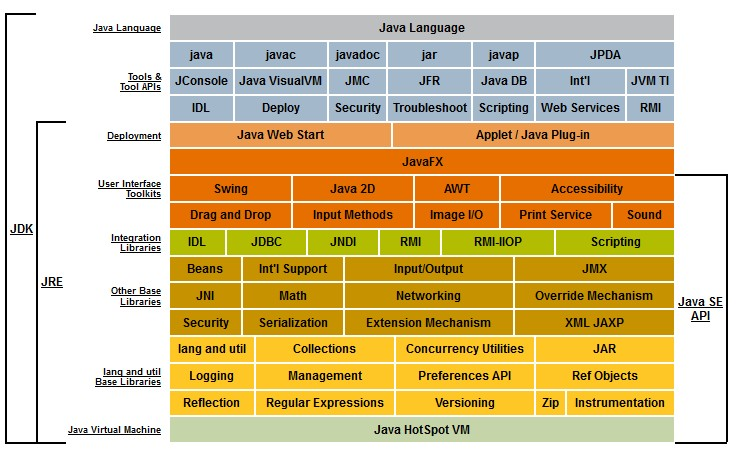# Number - Floating-point (system|notation) - (Float|Double) - Approximate numeric

The term floating point refers to the fact that the number's radix point can float, that is, it can be placed anywhere relative to the significant digits of the number.

They are fractional numbers written with a decimal point, like 1.970

The floating-point representation is the most widely representation of real numbers.

Floating point describes a numeral system for representing numbers that would be too large or too small to be represented as number. See also: wiki/Arbitrary-precision_arithmetic

The value 4.32682E-21F is an example of a float.

## Decimal point Position

The position of the decimal point is given by the exponent.

Floating-point numbers can have:

• a decimal point anywhere from the first to the last digit
• any number of digits after the decimal point
• no decimal point at all.

## Example

The number 1.25 has:

The number 5 has:

• a positive sign
• a mantissa value of 1.01 (in binary),
• and an exponent of 2 because the mantissa is multiplied by 4 (2 to the power of the exponent 2); 1.25 * 4 equals 5.

## Syntax and Properties

Floating-point numbers are made up of four components:

• Digits (normally only significant digit)
• The sign, which is positive or negative.
• The mantissa, which is a single-digit binary number followed by a fractional part.
• The exponent, which tells where the decimal point is located in the number represented.

### Sign

The sign is positive or negative

### Mantissa

The mantissa is a single-digit binary number followed by a fractional part.

For example:

In the SQL float or double data type , the precision gives the maximum length of the mantissa.

### Exponent

An exponent may optionally be used following the number to increase the range (for example, 1.777 e-20).

It tells where the decimal_point is located in the number.

## Documentation / Reference

Recommended PagesComputer - Floating-point (Representation and usage)

Computer representations of floating point numbers typically use a form of rounding to significant figures, but with binary numbers. The number of correct significant figures is closely related to the...Computer Number - Float64 (64-bit or double precision) floating-point number

Float64 is a floating point number with a 64bit precision. Float64 is also known as: 64-bit floating-point values, double precision floating-point 64-bit IEEE-754 floating-point or justdoublintegefloat...Java - Number

in Java. The abstract class Number is the superclass of platform classes representing numeric values that are convertible to the primitive types byte, double, float, int, long, and short. The...Number - Addition (or Sum, Sigma) or Total

The addition of all numbers in a set is called a total. Sum can be use as: an aggregate or a analytic function. It can follow the full syntax of the analytic function and in this way create It's...Number - Fixed-point numbers (exact numbers)

A fixed-point number represents a limited-precision rational number that may have a fractional part. These numbers are stored internally in a scaled-integer form, typically in binary but sometimes in...Number - NaN (Not A Number)

NaN, or Not a Number, is a value that returns when impossible things are asked in arithmetic -- like: divide zero by zero. or during the bad coercion NaN (paradoxically named not a number) is a special...
Number System - Real Number (Scalar) -

A real number is a value that represents a quantity along a line. They have been called real to distinguish them from imaginary number. imaginary roots of polynomials The real numbers include all...Number, Numeric, Quantity

A number is a mathematical object used to: count, label, and measure. Example: When we are interested in the total number of each species of tortoise, or how many individuals there are per square...Oracle Database - Floating-Point Number Datatype (BINARY_FLOAT, BINARY_DOUBLE)

Oracle provides two special datatype to store floating-point data: Datatype Storage Format BINARY_FLOAT single precision (32 bit) IEEE 754...Oracle Database - NUMBER Data Type

The NUMBER data type stores zero as well as positive and negative fixed numbers with absolute values from 1.0 x 10-130 to (but not including) 1.0 x 10126. If you specify an arithmetic expression whose...